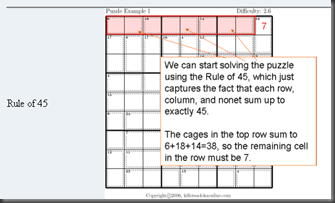## Thursday, 26 November 2009

### Trik RAHASIA MENYELESAIKAN PUZZLE SUDOKU

Mungkin anda tidak pernah kesulitan saat menyelesaikan sudoku pada level begginer, easy dan medium. tapi pada level lanjut, kening akan berkerut dan terjadi stagnasi seakan semua jurus sudah buntu. Kalu ini terjadi, sudah saatnnya anda membutuhkan jurus rahasia dalam menyelesaikan sudoku. berikut ini tip dan triknya:

sorry kalo treadnya masih dalam bahasa inggris (hitung-hitung belajar buka kamus lah dikit….)

#### Basic Solving Strategies

The following are the basic rules used to solve killer sudokus.
Rule of 1
This comes directly from the definition of sudoku. No region can contain any duplicate digits. In a sudoku region each digit appears exactly once. For example, if a digit appears in a row, it cannot be in any other cell in the row. Likewise, each digit can appear in a cage only once. If a digit is in a cage, it cannot appear in that cage again.
Rule of Necessity
This rule can be applied to sudoku regions (i.e., row, column, or nonet) or to a cage. In the former case, each region must contain all the digits one to nine. Thus, if all the digits but one appear in a row, the missing digit must appear in the empty cell.
Rule of 45
Each sudoku region (i.e., row, column, or nonet) contains the digits one through nine. Thus, each sudoku region has a total value of 45. If S is the sum of all the cages contained entirely in a region, then the cells not covered must sum to 45-S.
Rule of K
The Rule-of-k is an extension of the Rule-of-1. If there are k cells contained entirely in a region that contain exactly k different possible values, then no other cell in that region can contain any of those k values.
Sum Elimination
This strategy examines the different possible ways of making the sum of a cage. Reducing the number of different possible ways of making a sum, can often lead to a potential solution. There are many ways of reducing the number of sums. For example, if a 2-cage has a total of 3, 4, 16, or 17 there is only one combination of values that can be used. (3=2+1, 4=3+1, 16=9+7, and 17=9+8.) 3-cages with only 1 combination are: 6=1+2+3, 7=1+2+4, 23=9+8+6, 24=9+8+7. The sum calculator found in the online player page can be very handy.

ini sekedar contohnya :